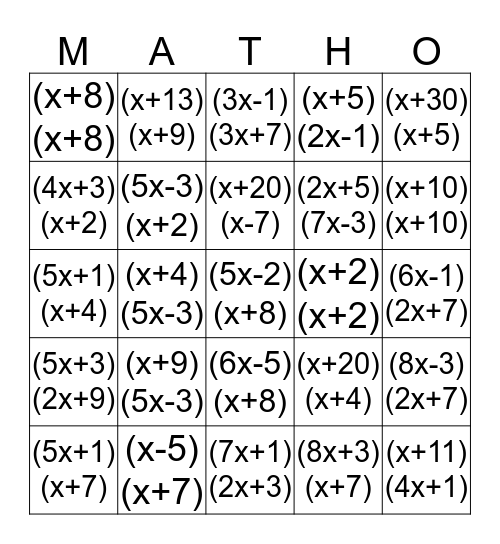# Binomial MultiplicationThis bingo card has 60 words: (x+4)(x-3), (x-5)(x+7), (2x-3)(x+3), (x+2)(x+2), (4x+1)(x+1), (x+2)(x+4), (5x-3)(x+2), (x+2)(3x+2), (x+5)(2x-1), (x+9)(x+1), (4x+3)(x+2), (x+6)(x+6), (x+20)(x-7), (9x+5)(x+1), (x+3)(2x+9), (x+8)(x+8), (x+4)(5x-3), (3x-1)(3x+7), (5x-1)(x+4), (x+10)(x+10), (5x+1)(x+4), (x+13)(x+9), (7x+1)(2x+3), (x+20)(x+4), (4x-1)(3x+7), (3x+7)(5x-3), (x+21)(x+6), (15x-2)(x+2), (2x+5)(7x-3), (x+15)(x+15), (2x+7)(3x+5), (x+2)(15x+2), (5x+3)(x+6), (x+7)(5x-1), (x+30)(x+5), (5x+1)(x+7), (x+7)(5x+2), (5x-2)(x+8), (x+9)(4x+3), (6x-1)(2x+7), (3x+7)(2x+9), (x+9)(5x-3), (6x-5)(x+8), (x+5)(9x-1), (x+11)(4x+1), (6x+7)(4x+3), (7x-1)(2x+7), (4x+7)(4x+5), (3x+5)(5x+8), (8x-3)(2x+7), (5x+3)(2x+9), (7x+3)(x+7), (5x+7)(9x-2), (4x+9)(2x+9), (8x+5)(3x+5), (x+5)(11x+1), (7x-3)(2x+9), (9x-5)(x+7), (8x+3)(x+7) and (5x+4)(5x+8).

## Play Online